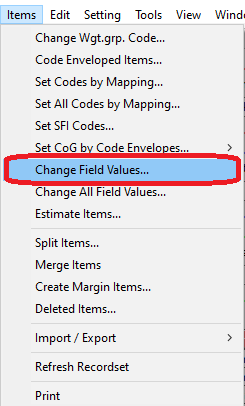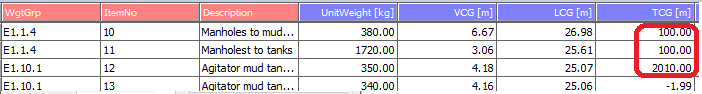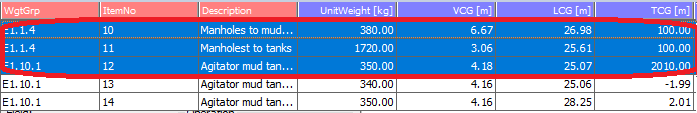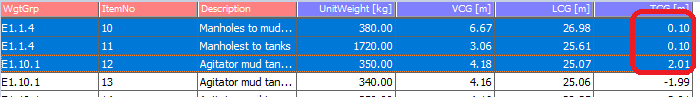Yes, you can use the Change Field Values... window in the Items window to do mathematical operations on multiple items.

First select the columns you would like to do a mathematical operation on. Then open the Change Field Values Window by clicking the Items menu and selecting Change Field Values... .To perform a mathematical operation on a numerical field in the selected columns you need to select that field in the Field selection (1). Then select add, subtract, multiply or divide in the operation field (2)  and then enter in the value you would like to add, subtract, multiply with or divide the selected field with in the Value field (3). When this is done click apply to execute the calculation. (4)Example: The QA-test discovered that TCG values were given in millimeters instead of meters for some items, correct the TCG values to be in meters.1. Select the columns containing the TCG with unit given in mm.2. Open the Change Field Values... window.3. Set field to be TCG.

4. Select the Operation to be Divide.

5. Set the value to 1000.

6. Click Apply.Now the selected columns have values given in meters in stead of mm.To read more check out the ShipWeight Online Help Manual.